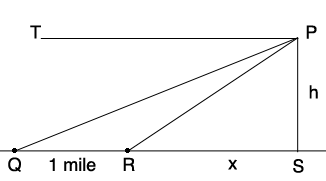SEARCH HOMEMath Central Quandaries & QueriesQuestion from Anna, a student: From the top of a hill, the angles of depression of two successive milestones on a level road, which leads straight away from the hill, are 5degrees and 15degrees respectively. Fine the height of the hill. Suggestion: BE is drawn perpendicular to AD. Find BE, then BD, finally CD. Thanks :)Hi Anna,

You didn't send a diagram so I am not sure what BE, AD etc. stand for so I drew my own diagram.P is the top of the hill and the two milestones are at Q and R. Since the angle of depression of the near milestone is 15 degrees the angle RPT has measure 15 degrees and thus the angle SPR has measure 75 degrees. The triangle SPR is a right triangle. What trig function relates the measure of the angle SPR and the lengths of the sides RS and SP? This gives you an equation relating $x$ and $h.$

Use the triangle SPQ to find another equation relating $x$ and $h.$ Solve for $x$ and $h.$

PennyMath Central is supported by the University of Regina and The Pacific Institute for the Mathematical Sciences.